#Calculus Worksheets

## Definite Integration Worksheets

Here is a graphic preview for all of the Definite Integration for Calculus Worksheets. You can select different variables to customize these Definite Integration for Calculus Worksheets for your needs. The Definite Integration for Calculus Worksheets are randomly created and will never repeat so you have an endless supply of quality Definite Integration for Calculus Worksheets to use in the classroom or at home. We have Fundamental Theorem of Calculus, Riemann Sum, summation properties, area, and mean value theorem worksheets.

Our Definite Integration for Calculus Worksheets are free to download, easy to use, and very flexible.

These Definite Integration for Calculus Worksheets are a good resource for students in high school.

Click here for a Detailed Description of all the Definite Integration for Calculus Worksheets.

## Quick Link for All Definite Integration for Calculus Worksheets

Click the image to be taken to that Definite Integration for Calculus Worksheets.

##### First Fundamental Theorem of Calculus Worksheets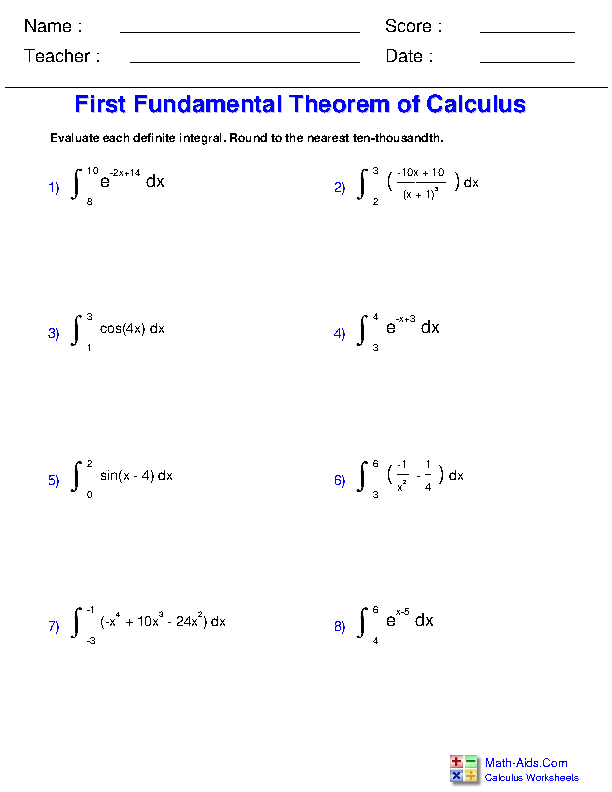##### Second Fundamental Theorem of Calculus Worksheets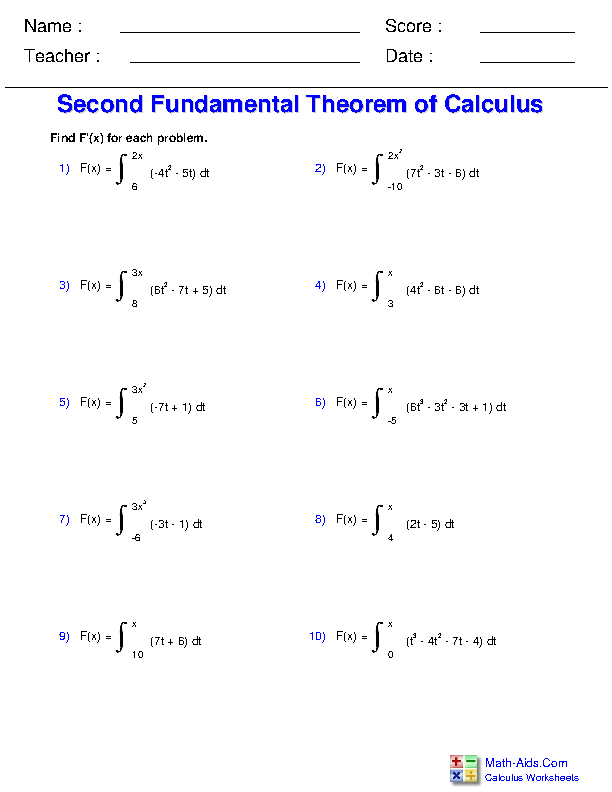##### Riemann Sum Tables Worksheets##### Summation Properties Worksheets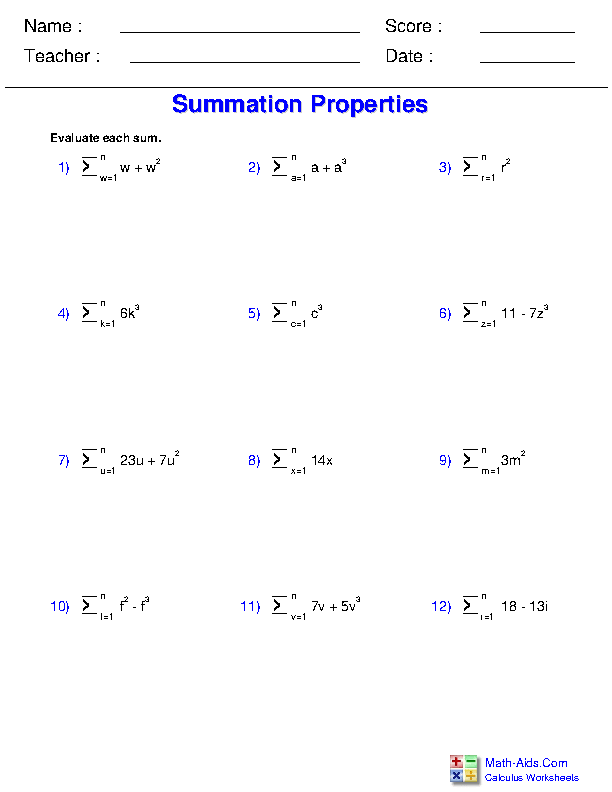##### Approximating the Area under a Curve Worksheets##### Substitution in Definite Integrals Worksheets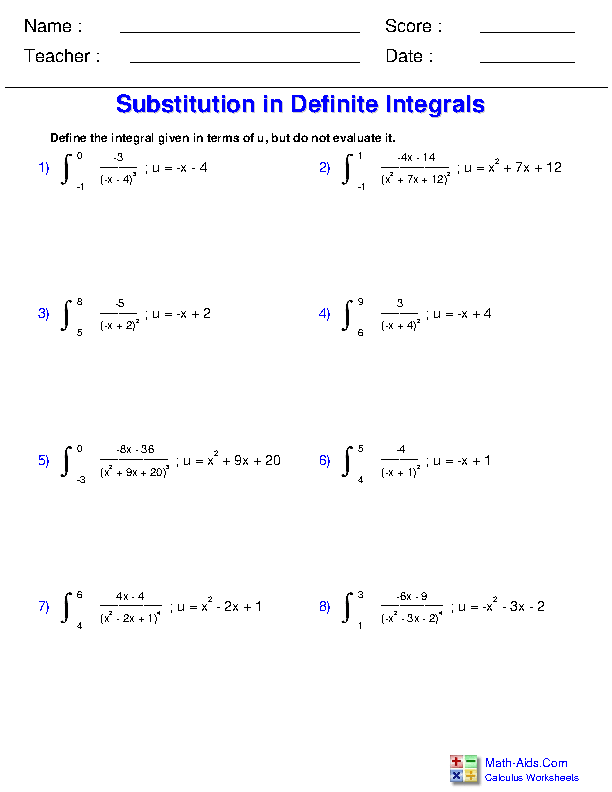##### Mean Value Theorem Worksheets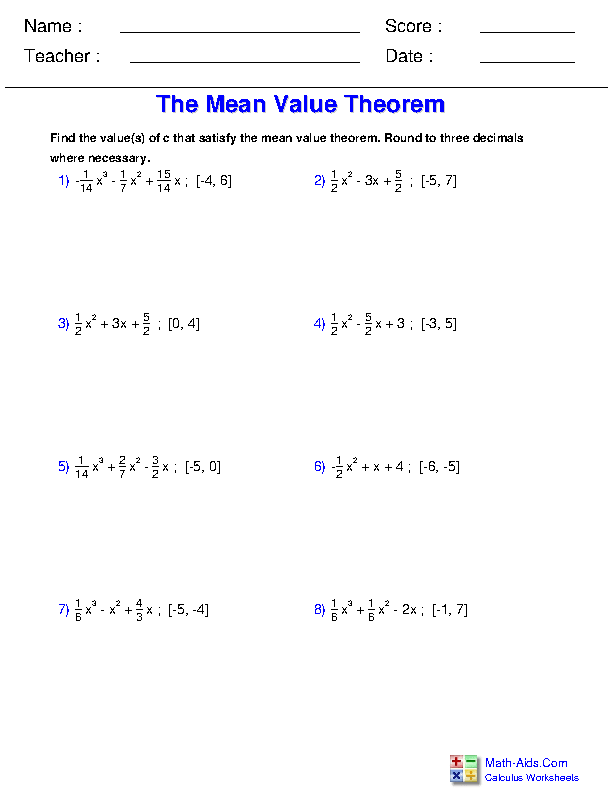Recommended Videos

## Detailed Description for All Definite Integration for Calculus Worksheets

First Fundamental Theorem of Calculus Worksheets
This Calculus - Definite Integration Worksheet will produce problems that involve using the first fundamental theorem of calculus to evaluate definite integrals.

Second Fundamental Theorem of Calculus Worksheets
This Distributive Property Worksheet will produce problems that involve using the second fundamental theorem of calculus to find derivatives.".

Riemann Sum Tables Worksheets
This Calculus - Definite Integration Worksheet will produce problems that involve drawing and solving Riemann sums based off of function tables.

Summation Properties Worksheets
This Calculus - Definite Integration Worksheet will produce problems that involve using summation properties to evaluate given sums.

Evaluating Expressions Worksheets
This Calculus - Definite Integration Worksheet will produce problems that involve approximating the area under a curve by drawing and calculating Riemann sums.

Substitution in Definite Integrals Worksheets
This Calculus - Definite Integration Worksheet will produce problems that involve using substitution in definite integrals to make them easier to evaluate.

Mean Value Theorem Worksheets
This Calculus - Definite Integration Worksheet will produce problems that involve finding a value that satisfies the mean value theorem, given a function and a domain.